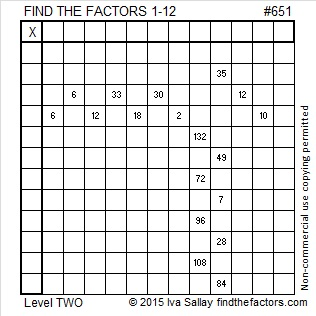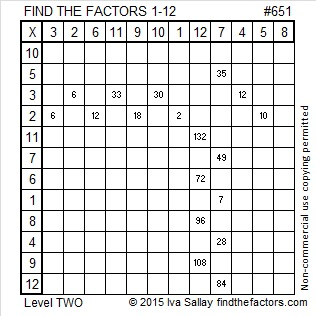# 651 and Level 2

21 x 31 = 651. Both of those factors are 5 away from their average, 26, so 651 is 25 less than 26² or 676.

The numbers in each of 651’s four factor pairs are odd, and the average of each and the distance each is from that average are both whole numbers. That means that 651 can be expressed as the difference of two squares four different ways. In this particular case the averages and distances generate four primitive Pythagorean triples with 651 as one of the legs:

• 26² – 5² = 651; primitive triple 260-651-701
• 50² – 43² = 651; primitive triple 651-4300-4349
• 110² – 107² = 651; primitive triple 651-23540-23549
• 326² – 325² = 651; primitive triple 651-211900-211901

651 is also a  pentagonal number.Print the puzzles or type the solution on this excel file: 12 Factors 2015-10-19

—————————————————————————————————

• 651 is a composite number.
• Prime factorization: 651 = 3 x 7 x 31
• The exponents in the prime factorization are 1, 1, and 1. Adding one to each and multiplying we get (1 + 1)(1 + 1)(1 + 1) = 2 x 2 x 2 = 8. Therefore 651 has exactly 8 factors.
• Factors of 651: 1, 3, 7, 21, 31, 93, 217, 651
• Factor pairs: 651 = 1 x 651, 3 x 217, 7 x 93, or 21 x 31
• 651 has no square factors that allow its square root to be simplified. √651 ≈ 25.5147.—————————————————————————————————This site uses Akismet to reduce spam. Learn how your comment data is processed.